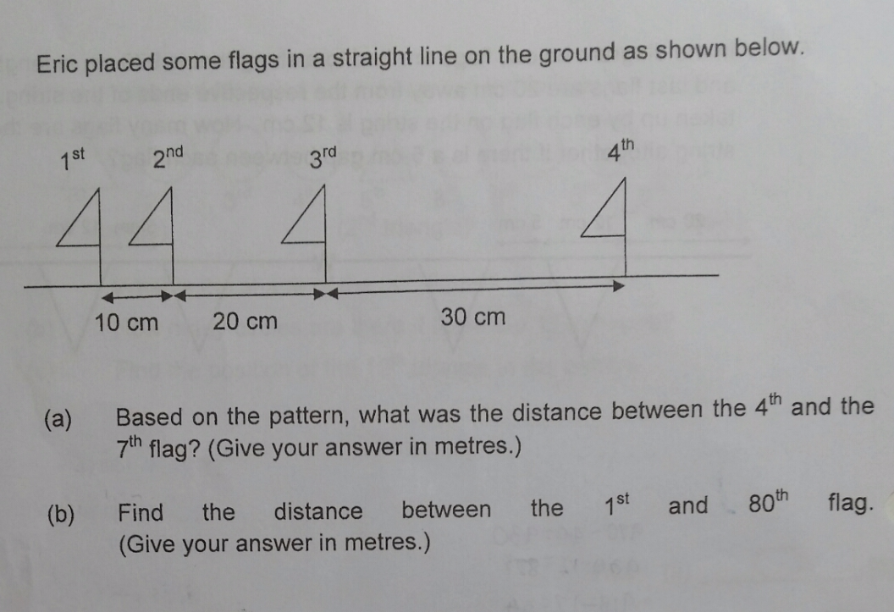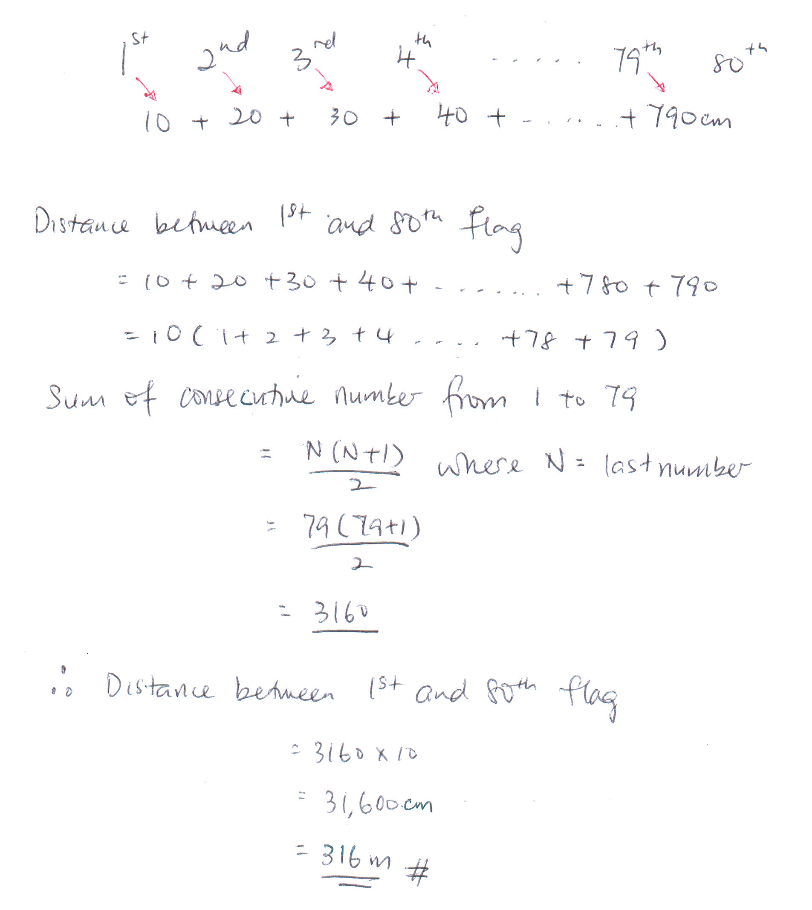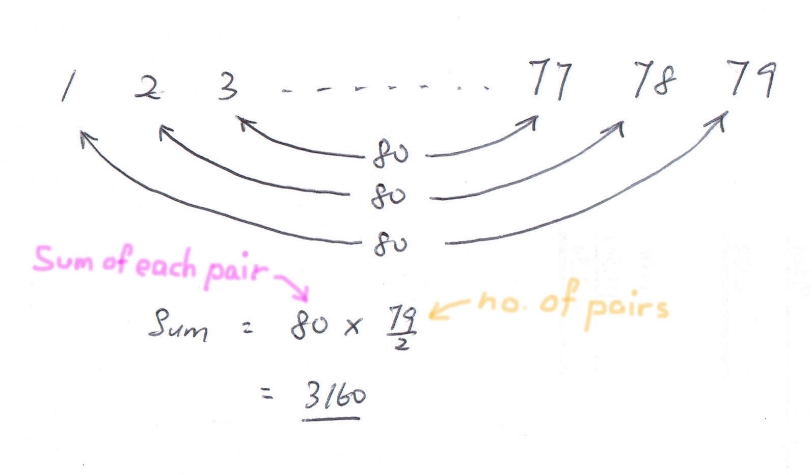# QuestionHi, can anyone help with question b please?  Thank you.

Source: Nan Chiau Primary

I would solve  (b) as follows ;

10 + 20 + 30 + … + 790 = (800 x 39) + 400 = 31600

or

10 + 20 + 30 + … + 790 = (790 x 39) + 790 = 31600

Ans : 316m.

0 Replies 1 LikeYou can use any method to find the sum of consecutive numbers from 10cm to 790cm ( in steps of 10 )